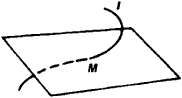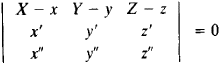# Osculating Plane

## osculating plane

[′äs·kyə‚lād·iŋ ′plān]
(mathematics)
For a curve C at some point p, this is the limiting plane obtained from taking planes through the tangent to C at p and containing some variable point p ′ and then letting p ′ approach p along C.

## Osculating Plane

The osculating plane of a curve l at a point M is the plane having contact of order n ≥ 2 with l at M. The oscillating plane can also be defined as the plane in the limitingFigure 1

position of the plane through three points of l as the points approach M.

From the standpoint of mechanics, the osculating plane can be characterized as the acceleration plane. As a mass point moves arbitrarily along l, the acceleration vector lies in the osculating plane.

Except for special cases, l usually penetrates the osculating plane at M (see Figure 1). If l is defined by the equations x = x(u), y = y(u), and z = z(u), the equation of the osculating plane is of the formHere, X, Y, and Z are the moving coordinates, and x, y, z, x’, y’, z’, x”, y”, and z” are computed at M. If all three coefficients of X, Y, and Z in the equation vanish, the osculating plane is undefined—it can coincide with any plane through the tangent. (See alsoDIFFERENTIAL GEOMETRY.)

### REFERENCE

Rashevskii, P. K. Kurs differentsial’noi geomelrii, 4th ed. Moscow, 1956.
References in periodicals archive ?
In this paper we have interested in Tzitzeica elliptic cylindrical curves in Minkowski 3-Space, more precisely we ask in what conditions a cylindrical curve is a Tzitzeica one, namely the function t [right arrow] [tau](t)/[d.sup.2(t) is constant, where d(t) is the distance from origin to the osculating plane of curve.
Further, we define the so called osculating plane of r spanned by the vectors r'(x) and r"(x) in the same point.
At each of the curve, the planes spanned by {T, N}, {T, B} and {N, B} are known respectively as the osculating plane, the rectifying plane and the normal plane.
Namely, rectifying, normal, and osculating planes of such curves always contain a particular point.

Site: Follow: Share:
Open / Close